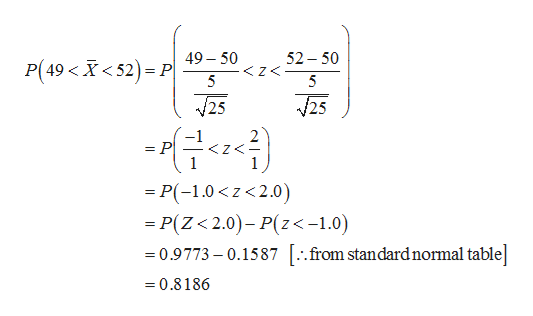# Give a normal population whose mean is 50 and whose standard deviation is 5, find the probability that a random sample of:4 has a mean between 49 and 5216 has a mean between 49 and 5225 has a mean between 49 and 52       Please do not solve using Microsoft Excel because my professor is not teaching using excel and I would like to see the steps involved in solving the equation.

Question

Give a normal population whose mean is 50 and whose standard deviation is 5, find the probability that a random sample of:

1. 4 has a mean between 49 and 52
2. 16 has a mean between 49 and 52
3. 25 has a mean between 49 and 52

Please do not solve using Microsoft Excel because my professor is not teaching using excel and I would like to see the steps involved in solving the equation.

check_circle

Step 1

1.

The probability that random sample of 4 has a mean between 49 and 52 is obtained below:

From the information, by central limit theorem for mean, follows a normal distribution with mean μ = 50 and standard deviation, 5 /sqrt (4) = 2.5.

Step 2

2.

The probability that random sample of 16 has a mean between 49 and 52 is obtained below:

From the information, by central limit theorem for mean, follows a normal distribution with mean μ = 50 and standard deviation, 5 /sqrt (16) =1.25.

Step 3

3.

The probability that random sample of 25 has a mean between 49 and 52 is obtained below:

From the information, by central li...help_outlineImage Transcriptionclose52 50 5 49-50 P(49 X<52) P 25 25 = P Z< =P(-10z2.0) P(Z<2.0)- P(z<-1.0) 0.9773- 0.1587 [..from standard normal table] =0.8186 fullscreen

### Want to see the full answer?

See Solution

#### Want to see this answer and more?

Solutions are written by subject experts who are available 24/7. Questions are typically answered within 1 hour.*

See Solution
*Response times may vary by subject and question.
Tagged in

### Other